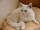Lenka and money

When Lenka bought pants from 1/3 of her savings shirt from 1/5 and 1/8 spent at the hairdresser, she is left 328 Kč.

How much money was saved? How much hairdresser cost?

Result

x =  960
k =  120

Solution:

x - 1/3 x - 1/5 x - k = 328
k = 1/8 x

15k-7x = -4920
8k-x = 0

k = 120
x = 960

Calculated by our linear equations calculator.

Leave us a comment of this math problem and its solution (i.e. if it is still somewhat unclear...):Be the first to comment!To solve this verbal math problem are needed these knowledge from mathematics:

Need help calculate sum, simplify or multiply fractions? Try our fraction calculator. Do you have a linear equation or system of equations and looking for its solution? Or do you have quadratic equation?

Next similar math problems:

1. Class 9.CProfessor collects money in the 9. C for a school trip. 2/3 of the collected amount was from girls and 1/4 from boys. The rest of 410 CZK went from the class fund. How much will the school trip cost in total?
2. CatOne-fifth of the monthly pocket money contributes Maros for food for his cat, half of the rest postpone for a new smartphone. The remaining € 8 spend. How much pocket money gets Maros a month?
3. Spain vs USASpain lost to the US by 4 goals. In the match total fell 10 goals. How many goals gave the Spain and how the United States?
4. Football match 4In a football match with the Italy lost 3 goals with Germans. Totally fell 5 goals in the match. Determine the number of goals of Italy and Germany.
5. Equation with fractionsSolve equation: ? It is equation with fractions.
6. Cleaning windowsCleaning company has to wash all the windows of the school. The first day washes one-sixth of the windows of the school, the next day three more windows than the first day and the remaining 18 windows washes on the third day. Calculate how many windows ha
7. FractionsThree-quarters of an unknown number are 4/5. What is 5/6 of this unknown number?
8. EquationSolve the equation: 1/2-2/8 = 1/10; Write the result as a decimal number.
9. One thirdIf 3/5 is 360, how much is 1/3?
10. Equation with xSolve the following equation: 2x- (8x + 1) - (x + 2) / 5 = 9
11. Trees - orchardThe 3/5 trees are apple trees and 1/3 of the trees are cherries. The remaining 5 trees are pear trees. How many trees are in the orchard.
12. Fraction + eqSolve following simple equation with fractions: -5/6(8+5b) = 75 + 5/3b
13. Eq-fracSolve the following equation with fractions: h + 1/3 =5/3
14. Unknown numberI think the number - its sixth is 3 smaller than its third.
15. EqnSolve equation with fractions: 2x/3-50=40+x/4
16. Simple equationSolve for x: 3(x + 2) = x - 18
17. Linear systemSolve this linear system (two linear equations with two unknowns): x+y =36 19x+22y=720